# Introduction to Logic Sections 1 1 1 2

• Slides: 46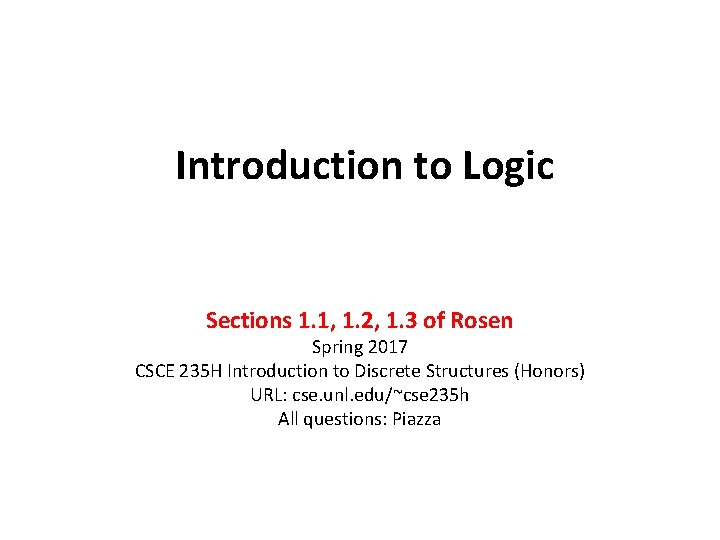Introduction to Logic Sections 1. 1, 1. 2, 1. 3 of Rosen Spring 2017 CSCE 235 H Introduction to Discrete Structures (Honors) URL: cse. unl. edu/~cse 235 h All questions: Piazza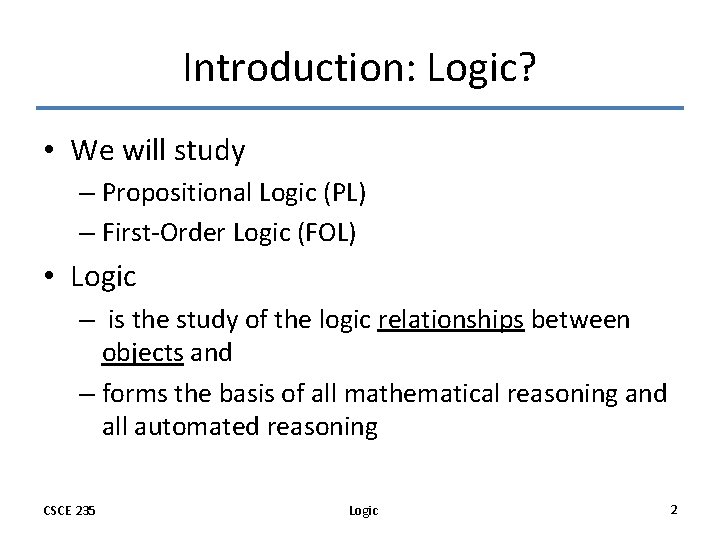Introduction: Logic? • We will study – Propositional Logic (PL) – First-Order Logic (FOL) • Logic – is the study of the logic relationships between objects and – forms the basis of all mathematical reasoning and all automated reasoning CSCE 235 Logic 2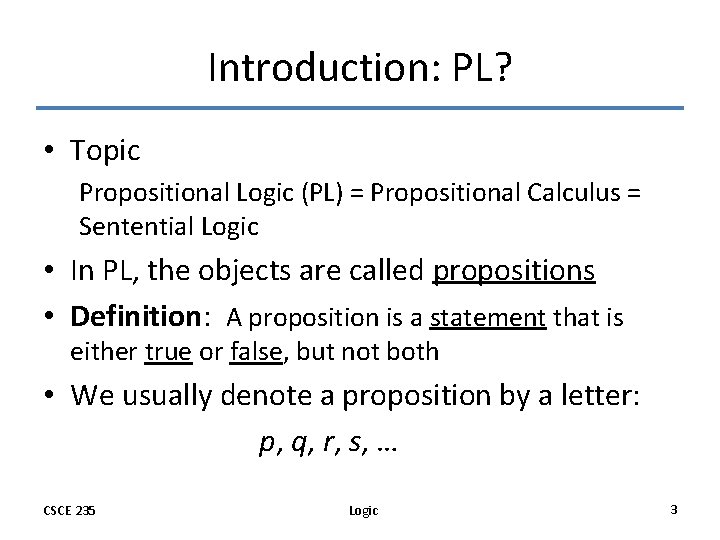Introduction: PL? • Topic Propositional Logic (PL) = Propositional Calculus = Sentential Logic • In PL, the objects are called propositions • Definition: A proposition is a statement that is either true or false, but not both • We usually denote a proposition by a letter: p, q, r, s, … CSCE 235 Logic 3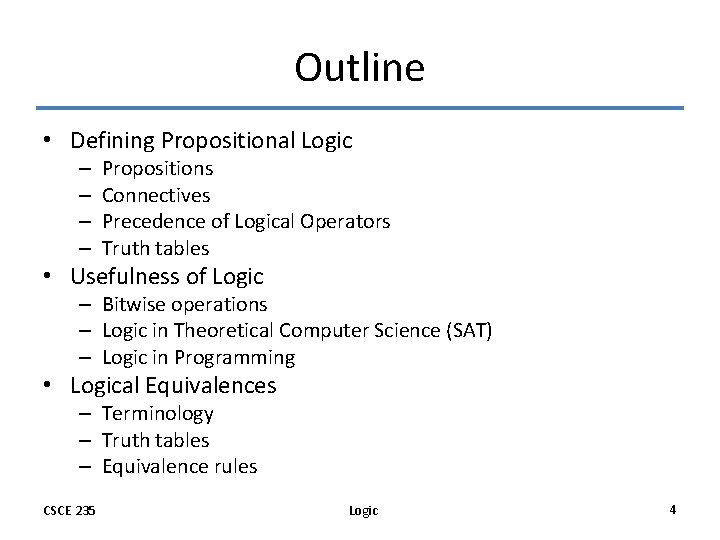Outline • Defining Propositional Logic – – Propositions Connectives Precedence of Logical Operators Truth tables • Usefulness of Logic – Bitwise operations – Logic in Theoretical Computer Science (SAT) – Logic in Programming • Logical Equivalences – Terminology – Truth tables – Equivalence rules CSCE 235 Logic 4Introduction: Proposition • Definition: The value of a proposition is called its truth value; denoted by – T or 1 if it is true or – F or 0 if it is false • Opinions, interrogatives, and imperatives are not propositions • Truth table p 0 1 CSCE 235 Logic 5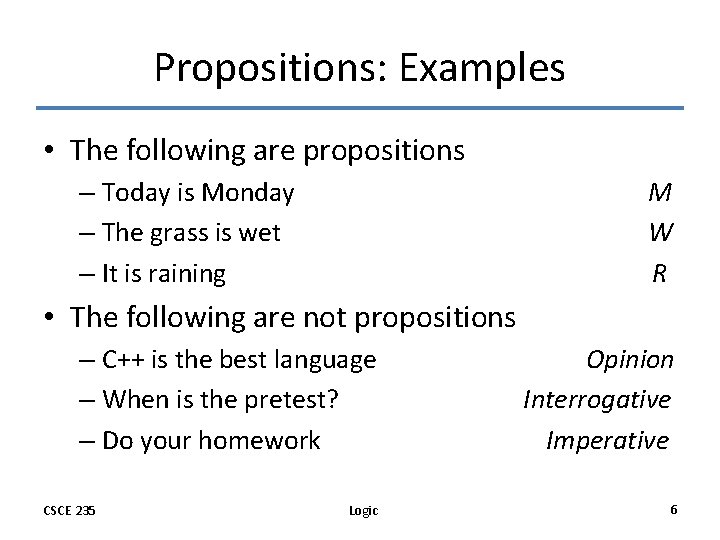Propositions: Examples • The following are propositions – Today is Monday – The grass is wet – It is raining M W R • The following are not propositions – C++ is the best language – When is the pretest? – Do your homework CSCE 235 Logic Opinion Interrogative Imperative 6Are these propositions? • 2+2=5 • Every integer is divisible by 12 – Alert: This statement is not a proposition: we cannot determine whether it is true or false. • Microsoft is an excellent company CSCE 235 Logic 7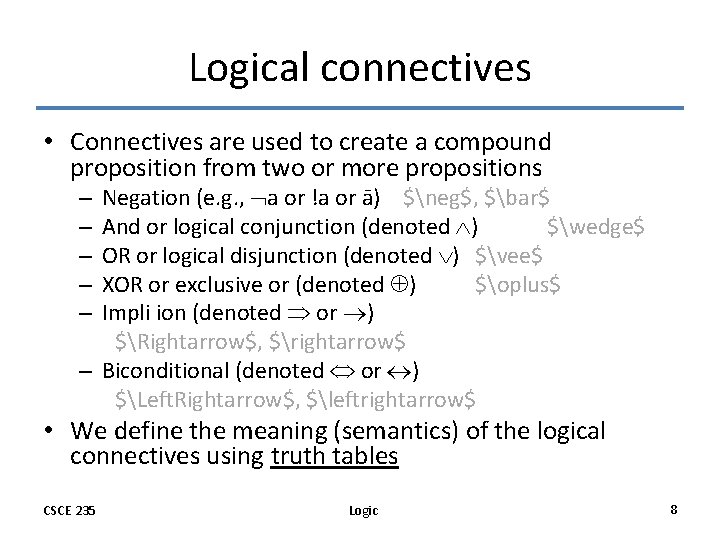Logical connectives • Connectives are used to create a compound proposition from two or more propositions Negation (e. g. , a or !a or ā) \$neg\$, \$bar\$ And or logical conjunction (denoted ) \$wedge\$ OR or logical disjunction (denoted ) \$vee\$ XOR or exclusive or (denoted ) \$oplus\$ Impli ion (denoted or ) \$Rightarrow\$, \$rightarrow\$ – Biconditional (denoted or ) \$Left. Rightarrow\$, \$leftrightarrow\$ – – – • We define the meaning (semantics) of the logical connectives using truth tables CSCE 235 Logic 8Precedence of Logical Operators • As in arithmetic, an ordering is imposed on the use of logical operators in compound propositions • However, it is preferable to use parentheses to disambiguate operators and facilitate readability p q r ( p) (q ( r)) • To avoid unnecessary parenthesis, the following precedences hold: – – – CSCE 235 Negation ( ) Conjunction ( ) Disjunction ( ) Implication ( ) Biconditional ( ) Logic 9Logical Connective: Negation • p, the negation of a proposition p, is also a proposition • Examples: – Today is not Monday – It is not the case that today is Monday, etc. • Truth table CSCE 235 p p 0 1 1 0 Logic 10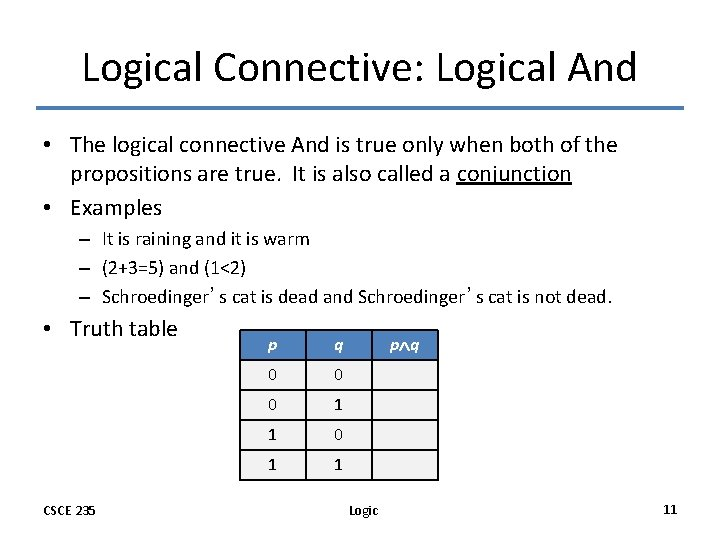Logical Connective: Logical And • The logical connective And is true only when both of the propositions are true. It is also called a conjunction • Examples – It is raining and it is warm – (2+3=5) and (1<2) – Schroedinger’s cat is dead and Schroedinger’s cat is not dead. • Truth table CSCE 235 p q 0 0 0 1 1 p q Logic 11Logical Connective: Logical OR • The logical disjunction, or logical OR, is true if one or both of the propositions are true. • Examples – It is raining or it is the second lecture – (2+2=5) (1<2) – You may have cake or ice cream • Truth table CSCE 235 p q 0 0 1 1 1 Logic p q 12Logical Connective: Exclusive Or • The exclusive OR, or XOR, of two propositions is true when exactly one of the propositions is true and the other one is false • Example – The circuit is either ON or OFF but not both – Let ab<0, then either a<0 or b<0 but not both – You may have cake or ice cream, but not both • Truth table CSCE 235 p q p q 0 0 0 1 1 1 Logic p q 13Logical Connective: Implication (1) • Definition: Let p and q be two propositions. The implication p q is the proposition that is false when p is true and q is false and true otherwise – p is called the hypothesis, antecedent, premise – q is called the conclusion, consequence • Truth table CSCE 235 p q p q 0 0 0 1 0 1 1 1 0 Logic p q 14Logical Connective: Implication (2) • The implication of p q can be also read as – If p then q – p implies q – If p, q – p only if q – q if p – q whenever p – q follows from p – p is a sufficient condition for q (p is sufficient for q) – q is a necessary condition for p (q is necessary for p) CSCE 235 Logic 15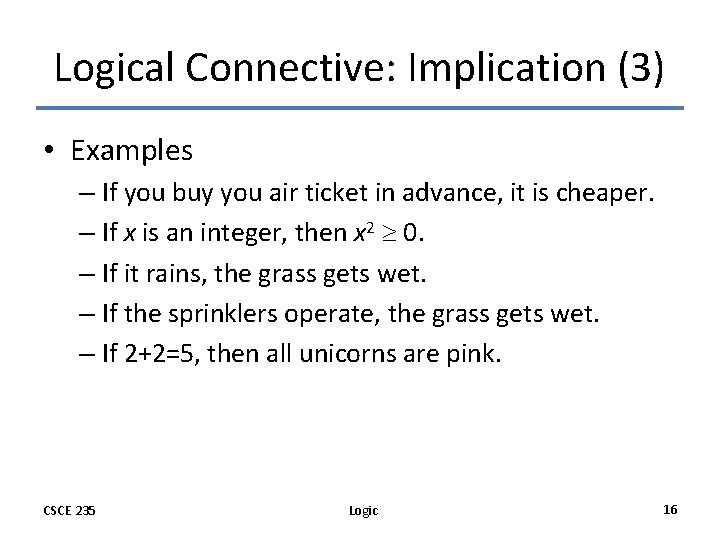Logical Connective: Implication (3) • Examples – If you buy you air ticket in advance, it is cheaper. – If x is an integer, then x 2 0. – If it rains, the grass gets wet. – If the sprinklers operate, the grass gets wet. – If 2+2=5, then all unicorns are pink. CSCE 235 Logic 16Exercise: Which of the following implications is true? • If -1 is a positive number, then 2+2=5 True. The premise is obviously false, thus no matter what the conclusion is, the implication holds. • If -1 is a positive number, then 2+2=4 True. Same as above. • If you get an 100% on your Midterm 1, then you will have an A+ on CSCE 235 False. Your grades homework, quizzes, Midterm 2, and Final, if they are bad, would prevent you from having an A+. CSCE 235 Logic 17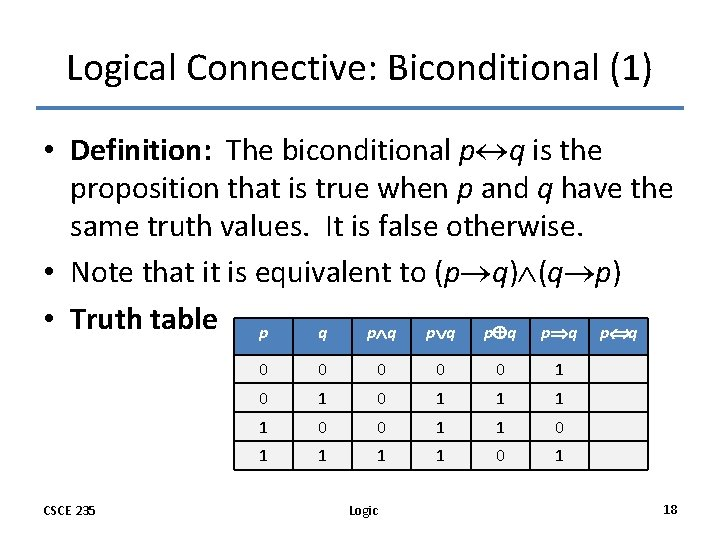Logical Connective: Biconditional (1) • Definition: The biconditional p q is the proposition that is true when p and q have the same truth values. It is false otherwise. • Note that it is equivalent to (p q) (q p) • Truth table p q p q p q CSCE 235 0 0 0 1 0 1 1 0 0 1 1 1 1 0 1 Logic 18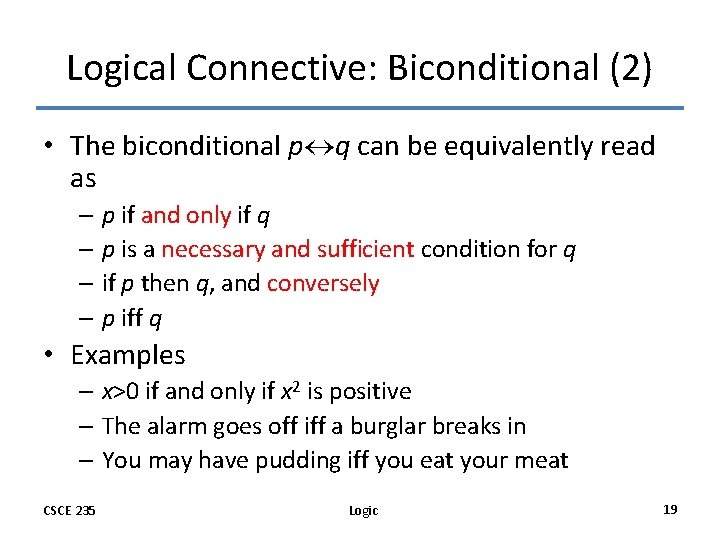Logical Connective: Biconditional (2) • The biconditional p q can be equivalently read as – p if and only if q – p is a necessary and sufficient condition for q – if p then q, and conversely – p iff q • Examples – x>0 if and only if x 2 is positive – The alarm goes off iff a burglar breaks in – You may have pudding iff you eat your meat CSCE 235 Logic 19Exercise: Which of the following biconditionals is true? • x 2 + y 2 = 0 if and only if x=0 and y=0 True. Both implications hold • 2 + 2 = 4 if and only if 2<2 True. Both implications hold. • x 2 0 if and only if x 0 False. The implication “if x 0 then x 2 0” holds. However, the implication “if x 2 0 then x 0” is false. Consider x=-1. The hypothesis (-1)2=1 0 but the conclusion fails. CSCE 235 Logic 20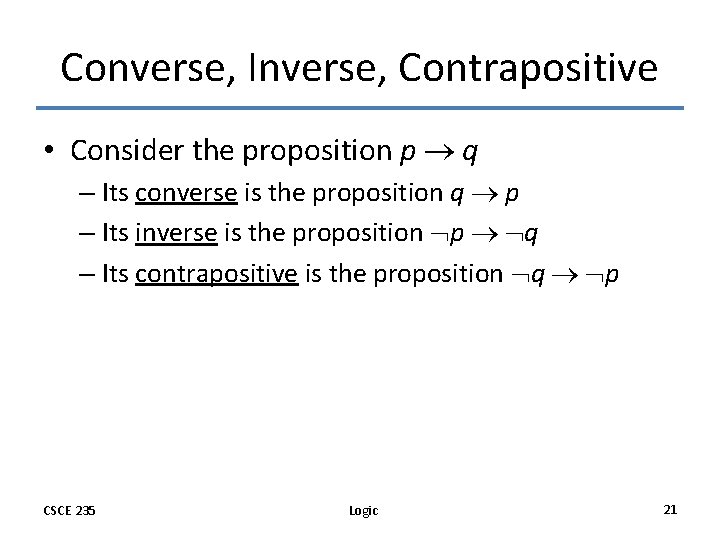Converse, Inverse, Contrapositive • Consider the proposition p q – Its converse is the proposition q p – Its inverse is the proposition p q – Its contrapositive is the proposition q p CSCE 235 Logic 21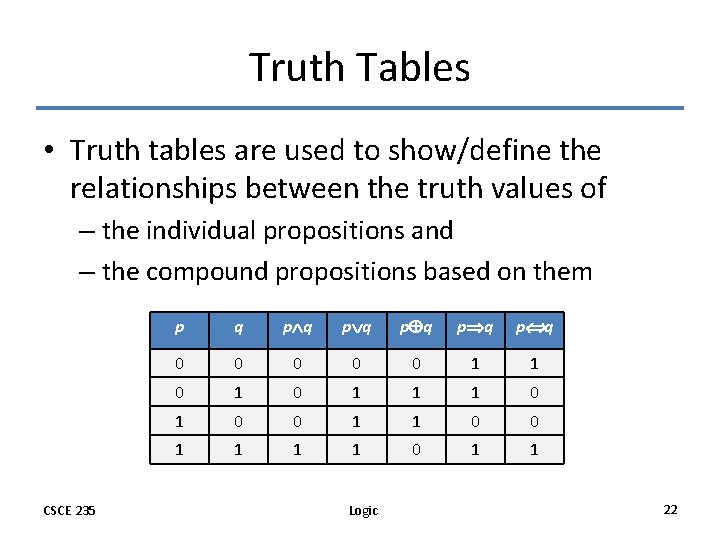Truth Tables • Truth tables are used to show/define the relationships between the truth values of – the individual propositions and – the compound propositions based on them CSCE 235 p q p q p q 0 0 0 1 1 1 0 0 1 1 0 1 1 Logic 22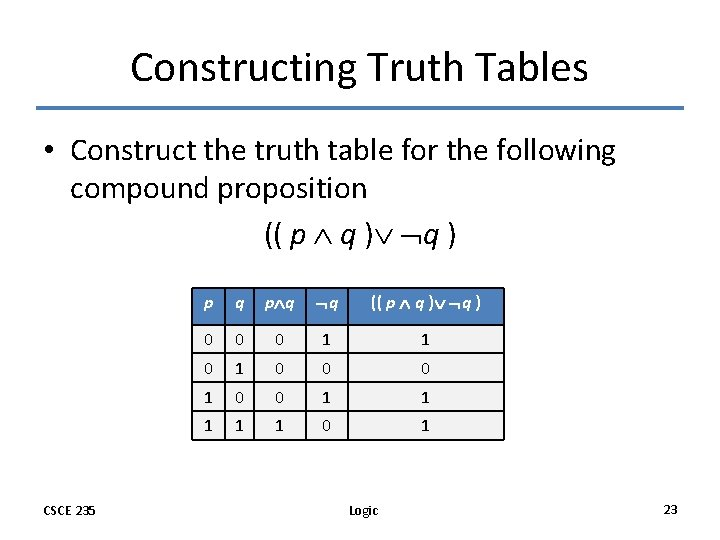Constructing Truth Tables • Construct the truth table for the following compound proposition (( p q ) CSCE 235 p q q (( p q ) 0 0 0 1 1 1 0 1 Logic 23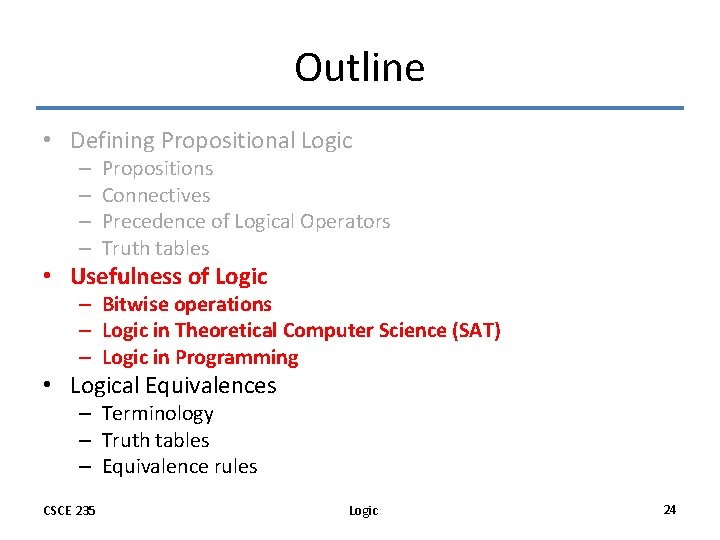Outline • Defining Propositional Logic – – Propositions Connectives Precedence of Logical Operators Truth tables • Usefulness of Logic – Bitwise operations – Logic in Theoretical Computer Science (SAT) – Logic in Programming • Logical Equivalences – Terminology – Truth tables – Equivalence rules CSCE 235 Logic 24Usefulness of Logic • Logic is more precise than natural language – You may have cake or ice cream. • Can I have both? – If you buy your air ticket in advance, it is cheaper. • Are there or not cheap last-minute tickets? • For this reason, logic is used for hardware and software specification – Given a set of logic statements, – One can decide whether or not they are satisfiable (i. e. , consistent), although this is a costly process… CSCE 235 Logic 25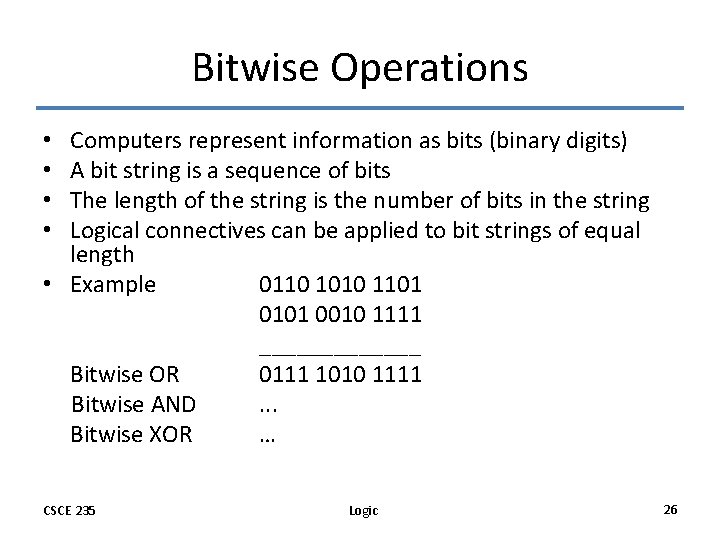Bitwise Operations Computers represent information as bits (binary digits) A bit string is a sequence of bits The length of the string is the number of bits in the string Logical connectives can be applied to bit strings of equal length • Example 0110 1010 1101 0010 1111 _______ Bitwise OR 0111 1010 1111 Bitwise AND. . . Bitwise XOR … • • CSCE 235 Logic 26Logic in TCS • What is SAT? SAT is the problem of determining whether or not a sentence in propositional logic (PL) is satisfiable. – Given: a PL sentence – Question: Determine whether or not it is satisfiable • Characterizing SAT as an NP-complete problem (complexity class) is at the foundation of Theoretical Computer Science. • What is a PL sentence? What does satisfiable mean? CSCE 235 Logic 27Logic in TCS: A Sentence in PL • A Boolean variable is a variable that can have a value 1 or 0. Thus, Boolean variable is a proposition. • A term is a Boolean variable • A literal is a term or its negation • A clause is a disjunction of literals • A sentence in PL is a conjunction of clauses • Example: (a b c d) ( b c) ( a c d) • A sentence in PL is satisfiable iff – we can assign a truth value – to each Boolean variables – such that the sentence evaluates to true (i. e. , holds) CSCE 235 Logic 28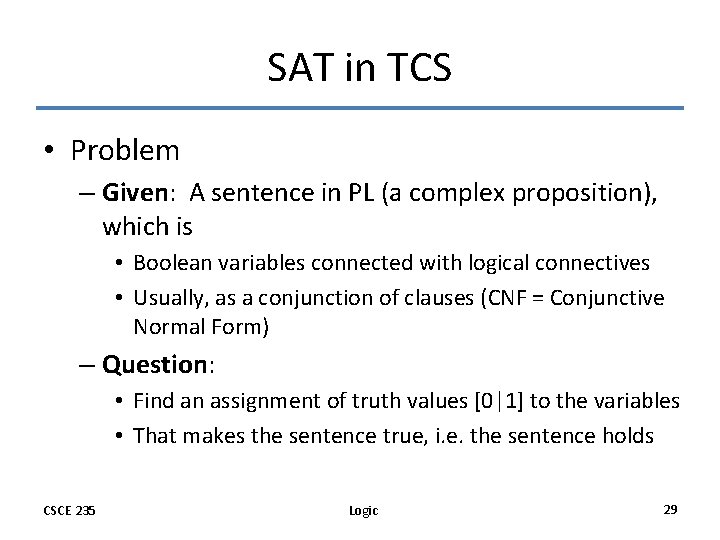SAT in TCS • Problem – Given: A sentence in PL (a complex proposition), which is • Boolean variables connected with logical connectives • Usually, as a conjunction of clauses (CNF = Conjunctive Normal Form) – Question: • Find an assignment of truth values [0|1] to the variables • That makes the sentence true, i. e. the sentence holds CSCE 235 Logic 29Logic in Programming: Example 1 • Say you need to define a conditional statement as follows: – Increment x if the following condition holds (x > 0 and x < 10) or x=10 • You may try: If (0<x<10 OR x=10) x++; • Can’t be written in C++ or Java • How can you modify this statement by using logical equivalence • Answer: If (x>0 AND x<=10) x++; CSCE 235 Logic 30Logic in Programming: Example 2 • Say we have the following loop While ((i<size AND A[i]>10) OR (i<size AND A[i]<0) OR (i<size AND (NOT (A[i]!=0 AND NOT (A[i]>=10))))) • Is this a good code? Keep in mind: – Readability – Extraneous code is inefficient and poor style – Complicated code is more prone to errors and difficult to debug – Solution? Comes later… CSCE 235 Logic 31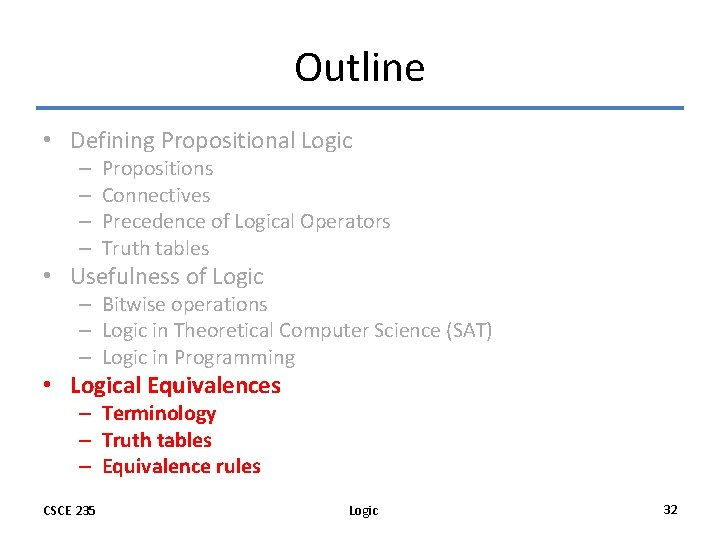Outline • Defining Propositional Logic – – Propositions Connectives Precedence of Logical Operators Truth tables • Usefulness of Logic – Bitwise operations – Logic in Theoretical Computer Science (SAT) – Logic in Programming • Logical Equivalences – Terminology – Truth tables – Equivalence rules CSCE 235 Logic 32Propositional Equivalences: Introduction • In order to manipulate a set of statements (here, logical propositions) for the sake of mathematical argumentation, an important step is to replace • one statement with • another equivalent statement • (i. e. , with the same truth value) • Below, we discuss – Terminology – Establishing logical equivalences using truth tables – Establishing logical equivalences using known laws (of logical equivalences) CSCE 235 Logic 33Terminology: Tautology, Contradictions, Contingencies • Definitions – A compound proposition that is always true, no matter what the truth values of the propositions that occur in it is called a tautology – A compound proposition that is always false is called a contradiction – A proposition that is neither a tautology nor a contradiction is a contingency • Examples – A simple tautology is p p – A simple contradiction is p p CSCE 235 Logic 34Logical Equivalences: Definition • Definition: Propositions p and q are logically equivalent if p q is a tautology. • Informally, p and q are equivalent if whenever p is true, q is true, and vice versa • Notation: p q (p is equivalent to q), p q, and p q • Alert: is not a logical connective \$equiv\$ CSCE 235 Logic 35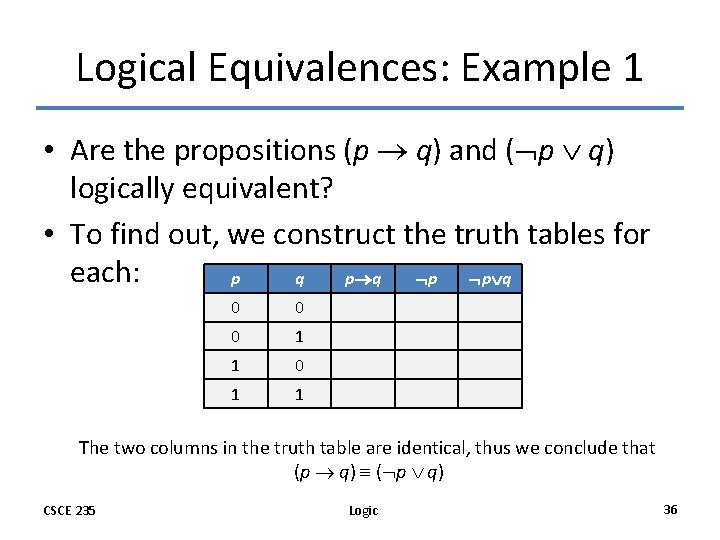Logical Equivalences: Example 1 • Are the propositions (p q) and ( p q) logically equivalent? • To find out, we construct the truth tables for each: p q p p q 0 0 0 1 1 The two columns in the truth table are identical, thus we conclude that (p q) ( p q) CSCE 235 Logic 36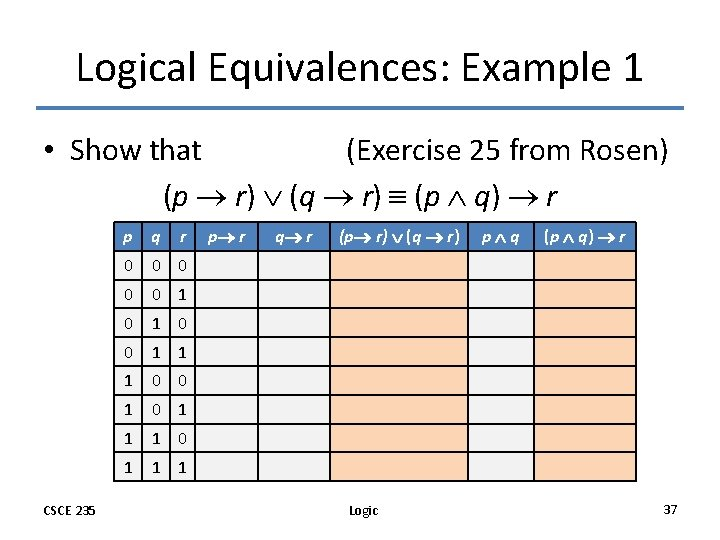Logical Equivalences: Example 1 • Show that (Exercise 25 from Rosen) (p r) (q r) (p q) r CSCE 235 p q r 0 0 0 1 1 1 0 0 1 1 1 p r q r (p r) (q r) Logic p q (p q) r 37Propositional Equivalences: Introduction • In order to manipulate a set of statements (here, logical propositions) for the sake of mathematical argumentation, an important step is to replace • one statement with • another equivalent statement • (i. e. , with the same truth value) • Below, we discuss – Terminology – Establishing logical equivalences using truth tables – Establishing logical equivalences using known laws (of logical equivalences) CSCE 235 Logic 38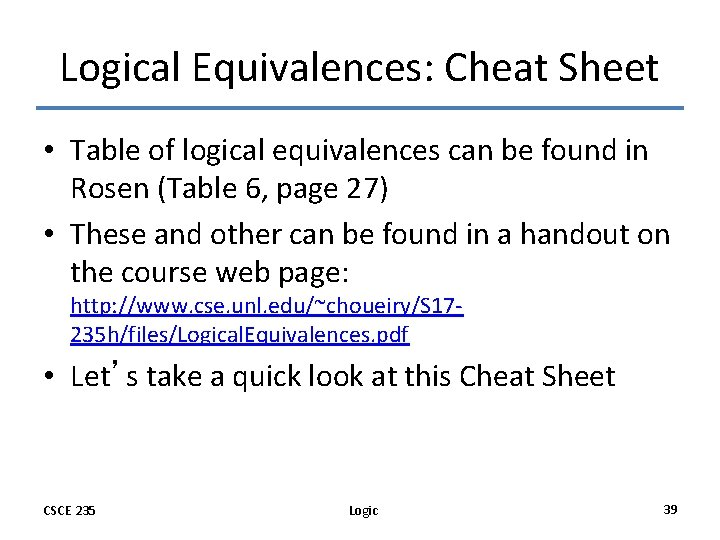Logical Equivalences: Cheat Sheet • Table of logical equivalences can be found in Rosen (Table 6, page 27) • These and other can be found in a handout on the course web page: http: //www. cse. unl. edu/~choueiry/S 17235 h/files/Logical. Equivalences. pdf • Let’s take a quick look at this Cheat Sheet CSCE 235 Logic 39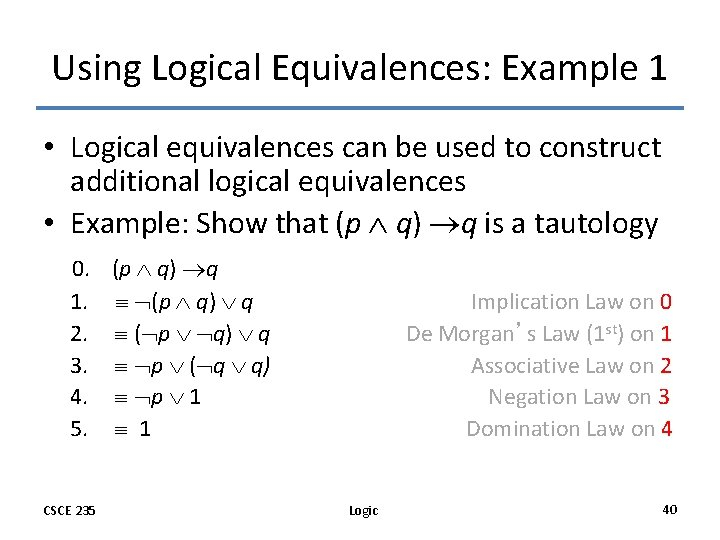Using Logical Equivalences: Example 1 • Logical equivalences can be used to construct additional logical equivalences • Example: Show that (p q) q is a tautology 0. (p q) q 1. (p q) q 2. ( p q) q 3. p ( q q) 4. p 1 5. 1 CSCE 235 Implication Law on 0 De Morgan’s Law (1 st) on 1 Associative Law on 2 Negation Law on 3 Domination Law on 4 Logic 40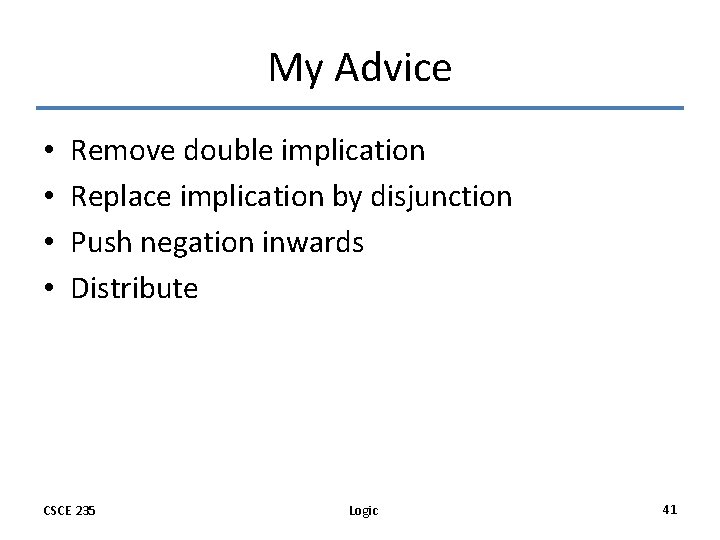My Advice • • Remove double implication Replace implication by disjunction Push negation inwards Distribute CSCE 235 Logic 41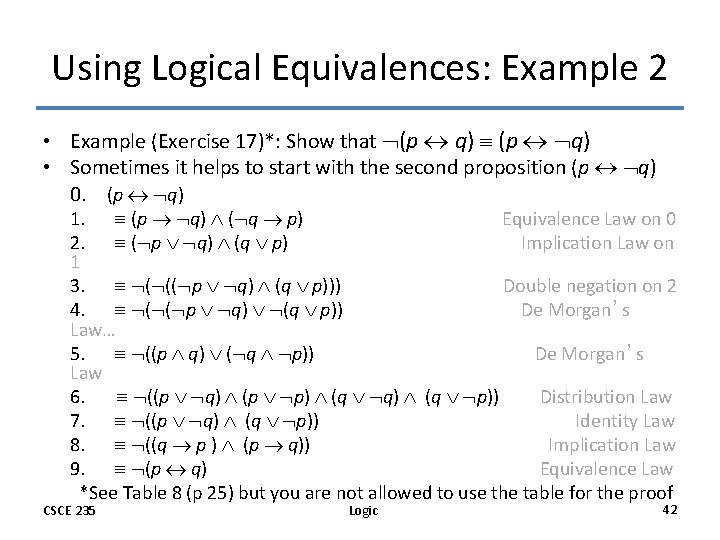Using Logical Equivalences: Example 2 • Example (Exercise 17)*: Show that (p q) • Sometimes it helps to start with the second proposition (p q) 0. (p q) 1. (p q) ( q p) Equivalence Law on 0 2. ( p q) (q p) Implication Law on 1 3. ( (( p q) (q p))) Double negation on 2 4. ( ( p q) (q p)) De Morgan’s Law… 5. ((p q) ( q p)) De Morgan’s Law 6. ((p q) (p p) (q q) (q p)) Distribution Law 7. ((p q) (q p)) Identity Law 8. ((q p ) (p q)) Implication Law 9. (p q) Equivalence Law *See Table 8 (p 25) but you are not allowed to use the table for the proof CSCE 235 Logic 42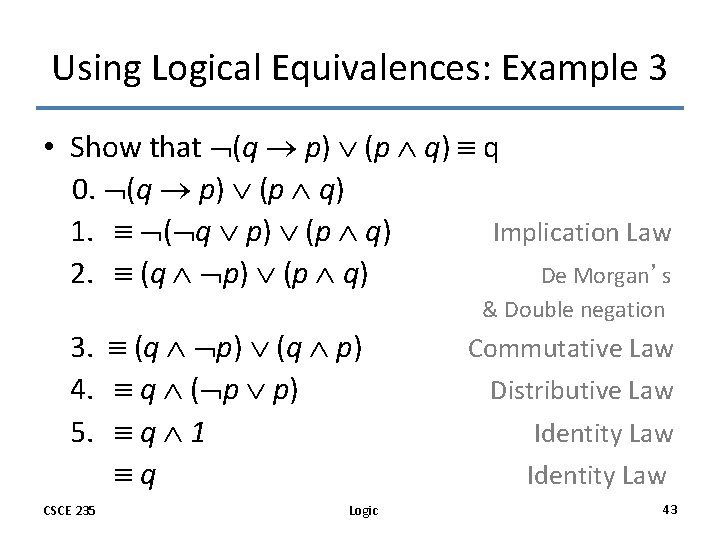Using Logical Equivalences: Example 3 • Show that (q p) (p q) q 0. (q p) (p q) 1. ( q p) (p q) Implication Law 2. (q p) (p q) De Morgan’s & Double negation 3. (q p) 4. q ( p p) 5. q 1 q CSCE 235 Logic Commutative Law Distributive Law Identity Law 43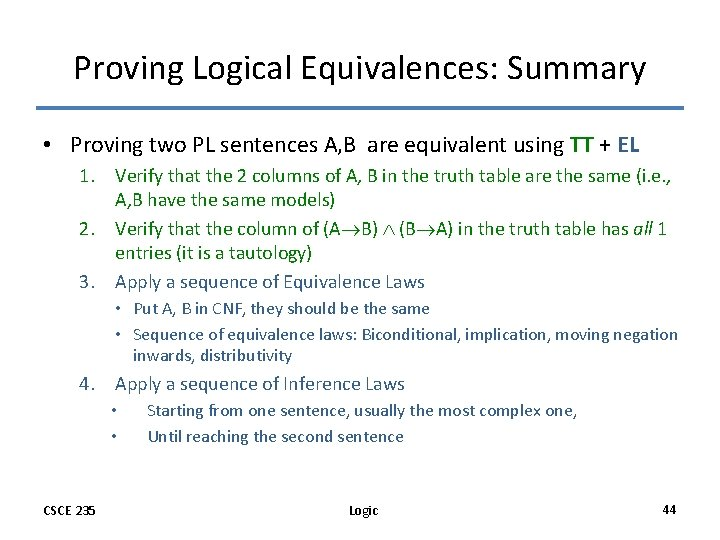Proving Logical Equivalences: Summary • Proving two PL sentences A, B are equivalent using TT + EL 1. Verify that the 2 columns of A, B in the truth table are the same (i. e. , A, B have the same models) 2. Verify that the column of (A B) (B A) in the truth table has all 1 entries (it is a tautology) 3. Apply a sequence of Equivalence Laws • Put A, B in CNF, they should be the same • Sequence of equivalence laws: Biconditional, implication, moving negation inwards, distributivity 4. Apply a sequence of Inference Laws • • CSCE 235 Starting from one sentence, usually the most complex one, Until reaching the second sentence Logic 44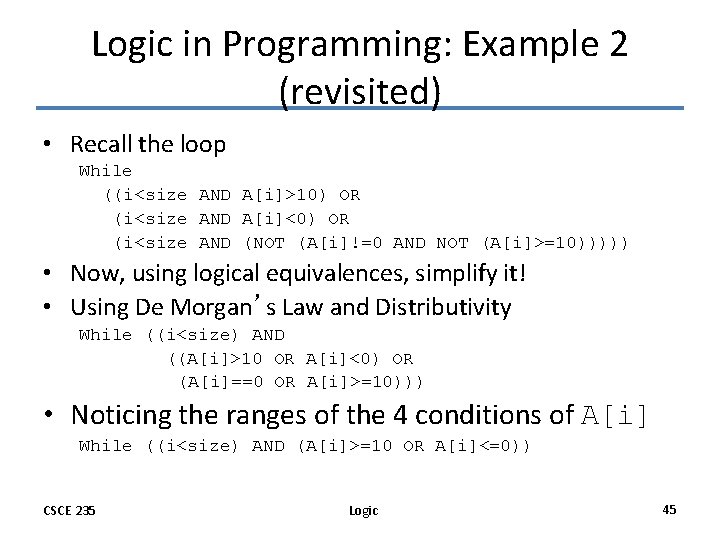Logic in Programming: Example 2 (revisited) • Recall the loop While ((i<size AND A[i]>10) OR (i<size AND A[i]<0) OR (i<size AND (NOT (A[i]!=0 AND NOT (A[i]>=10))))) • Now, using logical equivalences, simplify it! • Using De Morgan’s Law and Distributivity While ((i<size) AND ((A[i]>10 OR A[i]<0) OR (A[i]==0 OR A[i]>=10))) • Noticing the ranges of the 4 conditions of A[i] While ((i<size) AND (A[i]>=10 OR A[i]<=0)) CSCE 235 Logic 45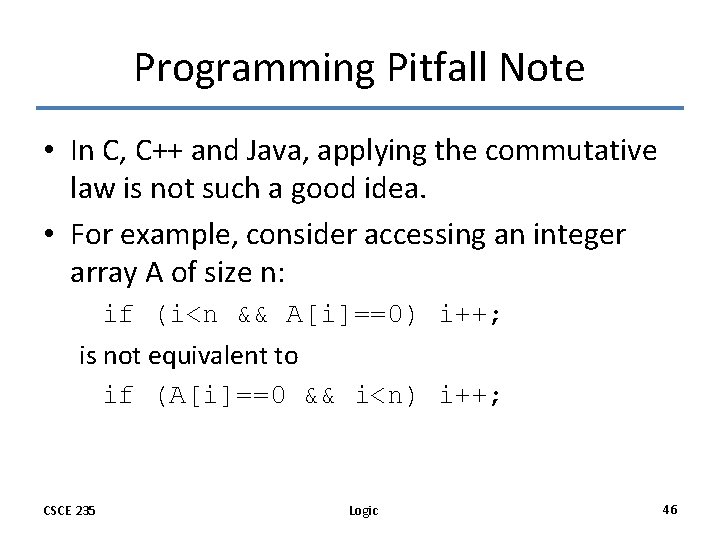Programming Pitfall Note • In C, C++ and Java, applying the commutative law is not such a good idea. • For example, consider accessing an integer array A of size n: if (i<n && A[i]==0) i++; is not equivalent to if (A[i]==0 && i<n) i++; CSCE 235 Logic 46